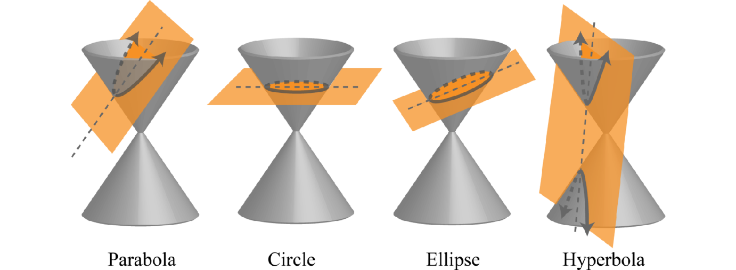# Classifying Conic Sections Notes

Author:
carattinim

## Conics in General Form

Ax2 + Bx2 + Dx + Ey + F ﻿ ﻿ ﻿ Rules to Remember:
• A and B cannot both equal zero - this would be the equation of a line
• if A = B, the conic is a circle
• if A or B = 0, the conic is a parabola
• if A is not equal to B and AB > 0, the conic is an ellipse
• if AB < 0, the conic is a hyperbola

## Relationships in Conic Sections﻿﻿Conic sections can be seen as "slices" of two inverted cones. The shapes created by these "slices" are the same as the shapes which you will graph using equations. The physical differences between sections are reflected in the equations of the sections.

1. y2 -